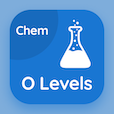Cambridge Online Courses (GCSE)

O Level Chemistry MCQs

O Level Chemistry MCQ PDF - Topics

# Chemical and Ionic Equations MCQ Quiz Online

Practice Chemical and Ionic Equations Multiple Choice Questions (MCQ), Chemical and Ionic Equations quiz answers PDF to learn o level chemistry online course for o level chemistry classes. Chemical Formulae and Equations Multiple Choice Questions and Answers (MCQs), Chemical and Ionic Equations quiz questions for ACT test. "Chemical and Ionic Equations MCQ" PDF Book: relative molecular mass, reactants, valency and chemical formula test prep for GRE subject tests.

"The formula of copper carbonate is" MCQ PDF: chemical and ionic equations with choices cuco3, cuco2, cucho3, and cucoh for ACT test. Learn chemical and ionic equations quiz questions for merit scholarship test and certificate programs for GRE prep classes.

## MCQs on Chemical and Ionic Equations Quiz

MCQ: The formula of copper carbonate is

CuCO3
CuCO2
CuCHO3
CuCOH

MCQ: To balance Al(OH)3 + HNO3 → Al(NO3)3 + H2O, number of Al(OH)3 molecules will be

1
2
3
4

MCQ: In Na2CO3.10H2O, percentage of water is

0.5009
0.1699
0.6507
0.6294

MCQ: A white smoke consisting of fine white solid particles is formed by

propane
butane
magnesium oxide
tin oxide

MCQ: Which one of them is a color gas?

methane
ethane
propane
butane

### More Topics from O Level Chemistry Course